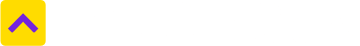Don't Miss out! Get notified of new homes as soon as they are posted

Notifications can be turned off anytime from browser settings.# Convert Square Inch to Square Feet

One Square Inch is equal to 0.006944458335 Square Feet
Select State
Select from unit
Square Inch
Select to unit
0.006944458335
Square Feet

## About Square Inch

A square inch is a unit of area measurement that equals to a square measuring one inch on each side. Among the symbols used to denote square inches are square in, sq inches, sq inch, sq in, etc. Used in the British Imperial and US system, this unit is also used for measuring small areas and is primarily used for making measurements on drawings like square centimeter. To calculate square inches in two-dimensional areas, the numbers can be totaled by the equation width × length, if the shape of the area is square or rectangle.

1 Square Inch is equal to

0.00064516 square meters

0.006 94 square feet

0.000 771 604 9382 square yards

6.4516 square centimetres

0.000 645 16 square metres

## Frequently Asked Questions

### What are the most common land measurement units in India?

Hectares, acres, square meters and square yards are some of the common land measurement units used across India. While Bigha and Marla are commonly used in the North, terms such as Cent, Guntha and Ground belong to the South. Depending upon the names of the units, their sizes also vary from State to State.

### How much is Square Inch in Square Feet terms?

One Square Inch is equal to 0.006944458335 Square Feet.

### How much is Square Feet in Square Inch terms?

One Square Feet is equal to 143.999712 Square Inch. Click here to convert

## Square Inch to other unit

Trusted by
Partners© 2012-21 Locon Solutions Pvt. Ltd.